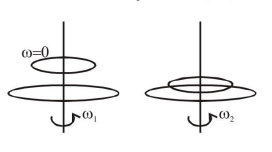# Solve this following

Question:

A circular disc of mass $M$ and radius $R$ is rotating about its axis with angular speed $\omega_{1}$. If another stationary disc having radius $\frac{\mathrm{R}}{2}$ and same mass $\mathrm{M}$ is dropped co-axially on to the rotating disc. Gradually both discs attain constant angular speed $\omega_{2}$. The energy lost in the process is $\mathrm{p} \%$ of the initial energy. Value of $p$ is

Solution:Let moment of inertia of bigger disc is $\mathrm{I}=\frac{\mathrm{MR}^{2}}{2}$

$\Rightarrow$ MOI of small disc $I_{2}=\frac{M\left(\frac{R}{2}\right)^{2}}{2}=\frac{I}{4}$

by angular momentum conservation

$\mathrm{I} \omega_{1}+\frac{\mathrm{I}}{4}(0)=\mathrm{I} \omega_{2}+\frac{\mathrm{I}}{4} \omega_{2} \Rightarrow \omega_{2}=\frac{4 \omega_{1}}{5}$

initial kinetic energy $\mathrm{K}_{1}=\frac{1}{2} \mathrm{I} \omega_{1}^{2}$

final kinetic energy $\mathrm{K}_{2}$

$=\frac{1}{2}\left(\mathrm{I}+\frac{\mathrm{I}}{4}\right)\left(\frac{4 \omega_{1}}{5}\right)^{2}=\frac{1}{2} \mathrm{I} \omega_{1}^{2}\left(\frac{4}{5}\right)$

$\mathrm{P} \%=\frac{\mathrm{K}_{1}-\mathrm{K}_{2}}{\mathrm{~K}_{1}} \times 100 \%=\frac{1-4 / 5}{1} \times 100=20 \%$